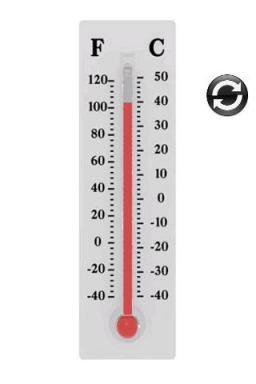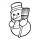# Outside temperature

The temperature outside was 57 degree Fahrenheit. During the next few hours it decreased by 18 degrees and then increased by 23 degrees. Find new temperature.

t =  62 °F

### Step-by-step explanation:

$t=57-18+23=62\text{°F}$Did you find an error or inaccuracy? Feel free to write us. Thank you!

Showing 1 comment:Math student
is 62## Related math problems and questions:

• The temperature 3The temperature is -12 degrees Fahrenheit. During the following 5 hours, the temperature decrease by 23 degrees Fahrenheit. What is the weather at 11 pm?
• Outdoor temperatureThe outdoor temperature was 8 degrees at midnight. The temperature declined 5 degrees during each of the next 3 hours. What was the temperature at 3am?
• At noonAt noon today it was 6°C outside, but it has increased 4°C since then. What is the temperature outside now?
• The temperature 6The temperature was 47°F on Thursday and 60°F on Friday. How much did the temperature rise?
• Temperature change 3At 2 PM, The temperature was 76 degrees Fahrenheit. At 8 PM, the temperature was 58 degrees Fahrenheit. What was the change in temperature?
• ThermometerThe thermometer showed -3°C in the morning. Then the temperature was increased by 1°C again increased by 1°C and then decreased by 1°C and then decreased by 4°C. Which terminal temperature thermometer shows?
• Temperature variationsToday's temperature was 80 degrees, and then the temperature dropped 10 degrees. Then it dropped 15 degrees again, then the next day, the temperature went up 2 degrees. What would the temperature be?
• OpheliaOphelia recorded the temperature of a cold store every two hours. 1. At 6 am, it was -4°C, and at 8 am, it was -1°C. By how much did the temperature rise? 2. The temperature went up by 5°C in the next two hours. .What was the temperature at 10 am?
• This morningThis morning it was 80 degrees F outside the temperature increased 12 degrees F. What is the temperature now?
• ThermometerThe thermometer showed -12 degrees Celsius in the morning then the temperature rises by 4 degrees and later again increased by 2 degrees at the evening has fallen by 5 degrees and then falls 3 degrees. What end temperature does the thermometer show?
• The bathDad poured 50 liters of water at 60°C into the tub. How much must cold water be added at 10°C to obtain a bath that has a temperature of 40°C?At a weather centre, the temperature at midnight was -2 degree Celsius and by noon it had raised 4 degree Celsius. What is the new temperature?Weather. At 5:00 am, the temperature was 28°C, and at noontime, it rose by 9°C. By 4:00 pm, it dropped 3°C, and by nighttime, it was 4°C less. What is the room temperature this time?The temperature was freezing, then it doubled, then it dropped 10 degrees, and then it increased by 40 degrees. The temperature is now 16 degrees. What was the starting temperature?The temperature at 6 am was 21 degrees Fahrenheit by noon, and the temperature dropped 32 degrees Fahrenheit. What was the temperature at noon?Starting temperature is 21°C, the highest temperature is 32°C. What is the change in temperature?On Friday, the temperature was 82°F. The temperature changed by –2°F on Saturday, and then it changed by 5°F on Sunday. What was the temperature on Sunday? How did the temperature change? Note we consider the mathematical problem formulated in this way to